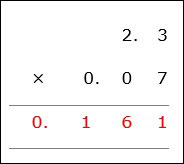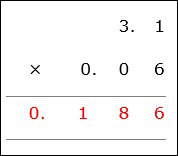# Decimal Multiplication

Decimal multiplication is similar to whole number multiplication.

Rules for Decimal Multiplication

• First we multiply the decimal numbers as if they were whole numbers by ignoring the decimal points.

• The number of digits after the decimal point in both the numbers are counted and added.

• In the final product, a decimal point is placed before that many digits from the right.

Multiply 0.07 × 2.3

### Solution

Step 1:

0.07 × 2.3

Multiplying without decimal points 7 × 23 = 161

Step 2:

The number of decimal places in 0.07 is 2

The number of decimal places in 2.3 is 1

Step 3:

So the product has three decimal places i.e.,0.07 × 2.3 = 0.161Multiply 0.06 × 3.1

### Solution

Step 1:

0.06 × 3.1

Multiplying without decimals 6 × 31 = 186

Step 2:

The decimal places in 0.06 is 2

The decimal places in 3.1 is 1

Step 3:

So the product has three decimal places i.e., 0.06 × 3.1 = 0.186